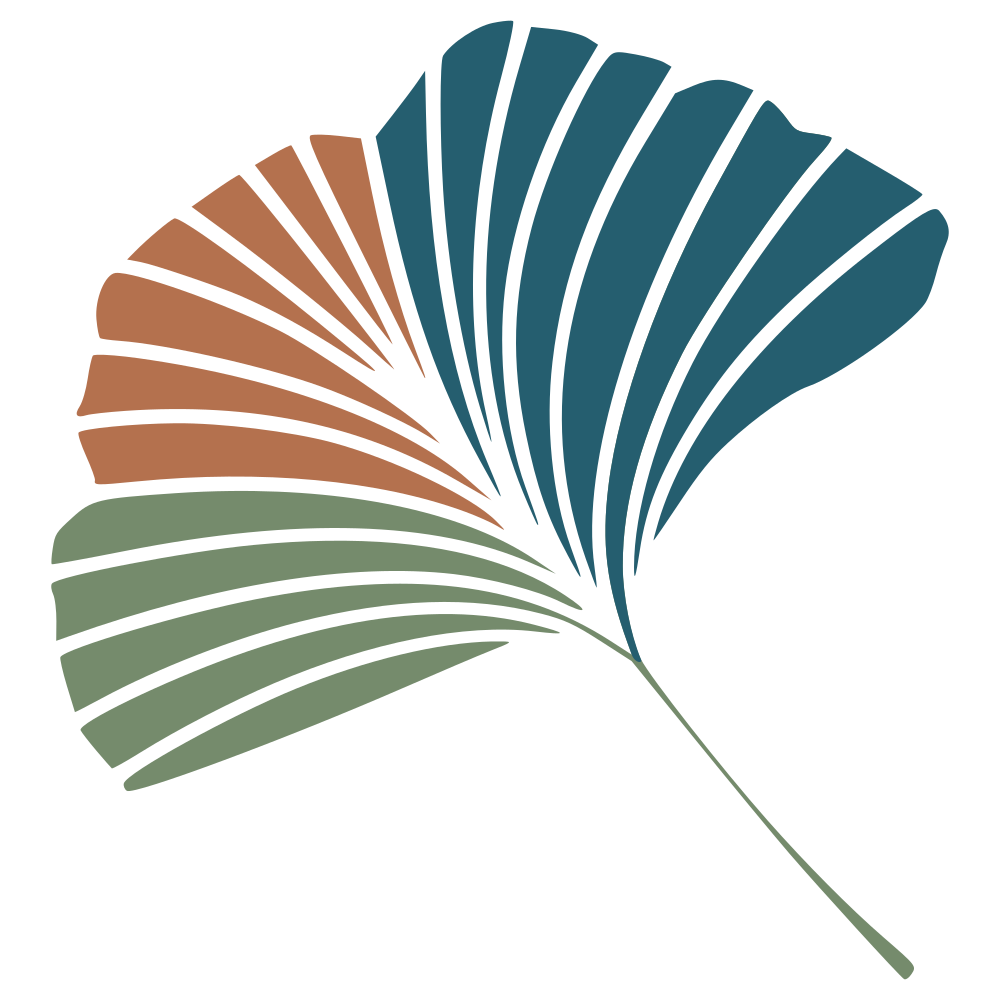# Quantifying species importance for network structure

A matrix decomposition approach

Do species matter? Debatable. But yesterday, at around 11pm and in a state of barely-held-together-chaos, I stumbled into something that I think makes sense, and that I wanted to formalize a little bit before deciding whether it is worth pursuing further. In short, maybe using Random Dot Graph Products gives us a way to measure the importance of a species in a network, in a way that is tied into how complex the network is overall. Let’s get started.

We will use a network from the web-of-life.es database, which is going to be large enough to do some analyses, but not so large that it’s too long to run for a blog post:

N = convert(BipartiteNetwork, web_of_life("M_PL_001"))

101×84 (String) bipartite ecological network (L: 361 - Bool)


What are are going to do is split the network in to using a Random Dot Product Graph – basically, this will return two matrices (left and right), the product of which gives a low-rank approximation of the network, at a rank we can define. The maximal rank we can use is the minimal richness of either side of the bipartite network.

This figure is an example of the actual network (first), and then approximations at increasingly deep ranks - the more information we take in, the more accurately we can approximate the shape of the network:In a sense, the left and right matrices returned by the RDPG are information about the “role” of species in relation to one another. So, can we use this to measure the importance of a species, specifically the importance it has in giving the network is overall structure?

Here is the general idea: if we remove a species, we can measure the extent to which it changes the resulting subspaces of the RDPG. If it has a strong importance, it should change the subspaces a lot. But more importantly, we can measure the disturbance for every rank of the subspace, as they are ordered. And so if a species is disturbing a low-rank a lot, it is presumably very important.

Specifically, for every species in the top leve (here, pollinators), we will calculate its left subspace, and compare the values to the left subspace of the entire network. For every rank, what we report is the squared error, so that larger values mean larger impact.

pollinators = species(N, dims=1)
rnk = 30
L, R = rdpg(N, rnk)
P = similar(L) # Perturbation matrix
for i in 1:richness(N, dims=1)
pset = deleteat!(collect(1:richness(N, dims=1)), i)
Lx, Rx = rdpg(N[pollinators[pset],:], rnk)
P[i,:] .= vec(sum((L[pset,:] .- Lx).^2.0, dims=1))
end


We can visualize the result of this analysis: every species is a column, and the light values means that the impact of the species at the rank is very high:The first rank remains unchanged, a few of the species have their maximal impacts at ranks 2 and 3, and then some other species have their maximal impact later on. We can extract two informations for this: what is the rank giving the maximal impact, and then what is the maximal impact reached at this rank?

peaks = mapslices(findmax, P; dims=2);
peak_at = [p for p in peaks];
peak_v = [p for p in peaks];


To get a complete picture of the situation, we can plot the peak on each perturbation for all top-level species in the network:This is nice, isn’t it? The species that affect the network early (low rank) have a strong impact, and the species that affect the network later (high rank) have a lower impact. We can complete this by looking at the cumulative total impact:

total_impact = sum(P; dims=2);


Let’s have a look at whether species that affect the network early have more impact:And now for the big question – is this just degree? Not it isn’t!Species with a larger degree do not necessarily have a larger impact on the network. One last thing I think should be noted is that the effect seems to be relatively constant aross ranks. If we compare the previous results (using the first 30 ranks), to the same simulations using the first 10, we can see this fairly clearly:In conclusion, what I like about this approach is that it can tell us something about how much a single species is responsible for the overall structure of the network. This is disconnected from any specific measure like nestedness, and even apparently disconnected from the degree of the node in question. This is probably something I will dig deeper into at some point.